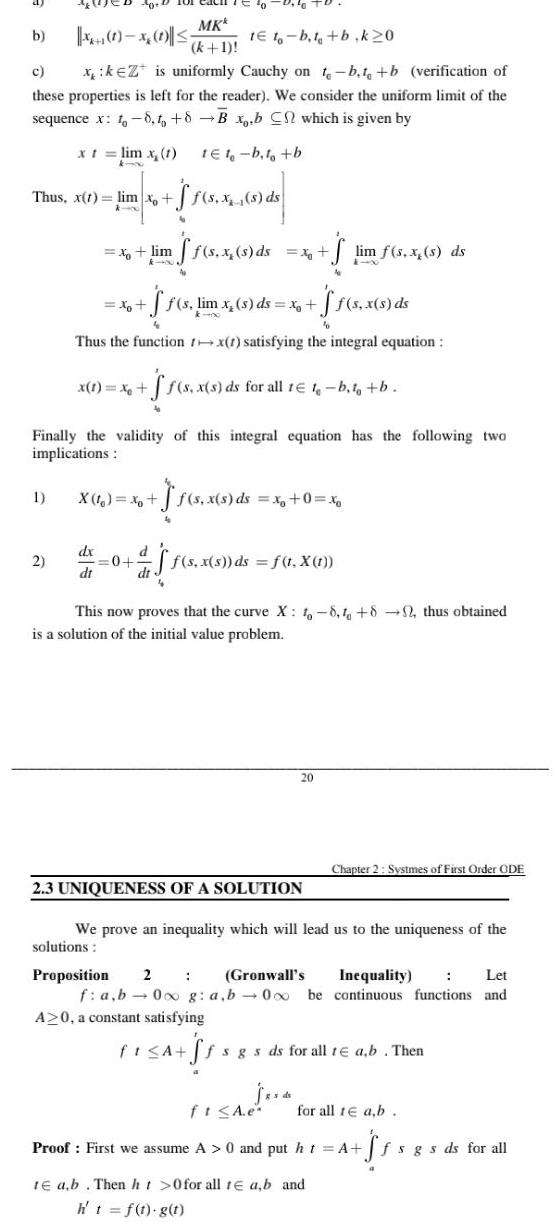Question:

# b 1 to b t b k20 c xkZ is uniformly Cauchy on t b t b

Last updated: 9/19/2023b 1 to b t b k20 c xkZ is uniformly Cauchy on t b t b verification of these properties is left for the reader We consider the uniform limit of the sequence x 1 8 to 8 B x b C which is given by x t lim x 1 Thus x t lim xo k 00 40 D 1 0 1 2 x lim x dx dt x 1 x MKk k 1 to 0 10 X 1 x 0 1e1 b t b ff s lim x s ds x Thus the function rx 1 satisfying the integral equation dt f s x s ds ff s x s ds Finally the validity of this integral equation has the following two implications x ff s x s ds for all t t b t tb f s x s ds x 0 x ff s x s ds lim f s x s ds f s x s ds f t X t 20 2 3 UNIQUENESS OF A SOLUTION This now proves that the curve X 1 8 1 82 thus obtained is a solution of the initial value problem s ds Chapter 2 Systmes of First Order ODE We prove an inequality which will lead us to the uniqueness of the solutions Proposition 2 Gronwall s Inequality Let f a b 0 g a b 000 be continuous functions and A 0 a constant satisfying fi A ff fsgs ds for all te a b Then ft A e Proof First we assume A 0 and put ht A te ab Then ht 0 for all te a b and h t f t g t for all 1 a b Sf fsgs ds for all